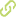# Increasing the training speed of SVM, the Zoutendijk algorithm case Chapter in Scopus•
• Overview
•
•
• View All
•

### Abstract

• The Support Vector Machine (SVM) is a well known method used for classification, regression and density estimation. Training a SVM consists in solving a Quadratic Programming (QP) problem. The QP problem is very resource consuming (computational time and computational memory), because the quadratic form is dense and the memory requirements grow square the number of data points. The support vectors found in the training of SVM's represent a small subgroup of the training patterns. If an algorithm could make an approximation beforehand of the points standing for support vectors, we could train the SVM only with those data and the same results could be obtained as trained using the entire data base. This paper introduces an original initialization by the Zoutendijk method, called ZQP, to train SVM's faster than classical ones. The ZQP method first makes a fast approximation to the solution using the Zoutendijk algorithm. As result of this approximation, a reduced number of training patterns is obtained. Finally, a QP algorithm makes the training with this subset of data. Results show the improvement of the methodology in comparison to QP algorithm and chunking with QP algorithm. The ideas presented here can be extended to another problems such as resource allocation, considering that allocation as a combinatorial problem, that could be solved using some artificial intelligent technique such as Genetic algorithms or simulated annealing. In such approach ZQP would be used as a measure for effective fitness. © Springer-Verlag Berlin Heidelberg 2005.

### Publication date

• December 1, 2005